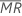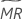# Estimating sigma for the I chart and the MR chart

The process standard deviation is also called sigma, or σ. If you enter an historical value for sigma, then Minitab uses the historical value. Otherwise, Minitab uses one of the following methods to estimate sigma from the data.

## Average moving range method

The average moving range,, of length w is given by the following formula:

where MRi is the moving range for observation i, calculated as follows:

Minitab usesto calculate Smr, which is an unbiased estimate of σ:

### Notation

TermDescription
nnumber of observations
w length of the moving range. The default is 2.
d2() value of unbiasing constant d2 that corresponds to the value specified in parentheses.

## Median moving range method

The median moving range,, of length w is given by the following formula:

where MRi is the moving range for observation i, calculated as follows:

Minitab usesto calculate Smr, which is an unbiased estimate of σ:

### Notation

TermDescription
nnumber of observations
w length of the moving range. The default is 2.
d4() value of unbiasing constant d2 that corresponds to the value specified in parentheses.
By using this site you agree to the use of cookies for analytics and personalized content.  Read our policy